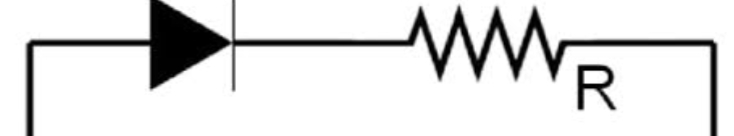### Diode Configuration

IDEAL Diode:

When diode in forward biased its act as short circuit when diode is received biased it act as open circuit in forward biased voltage across diode is zero bit current is non zero positive value and in reverse biased condition voltage  across Diode is negative and current through it is zero.

Practice Diode:

In practical diode when its in forward biased small voltage is present across the Diode called as cut in voltage which  is 0.7 for silicon and 0.3 for germanium and current through Diode is positive value which is varies from 0A to infinity in reverse biased condition small current will flows through Diode called minority current and voltage across it will Be negative.

Numericals:

1. For each of the following circuit find the value of Id, Vd  and also find the whether the Diode  is on or off assuming diode to  be on.

2k ohm

5V2V

Solution: Assuming diode to be ON

Applying KVL

5v-Id*2k-2v=0

Id=(5-2)/2

Id= 1.5 mA

The value of current is postive and greater than zero.

So assumption correct and Id=1.5 mA. And voltage is zero.

2k ohm

1.    2v5V

Solution: Assuming diode to be ON

Applying KVL

2V-Id-5V=0

Id=-1.5mA

The value of current is negative which is -1.5 mA

So assumption is wrong

So consider diode to be off

Now

Apply  KVL

Vd=-3V

Value of Vd is negative which is -3V and current is zero

So diode is off.